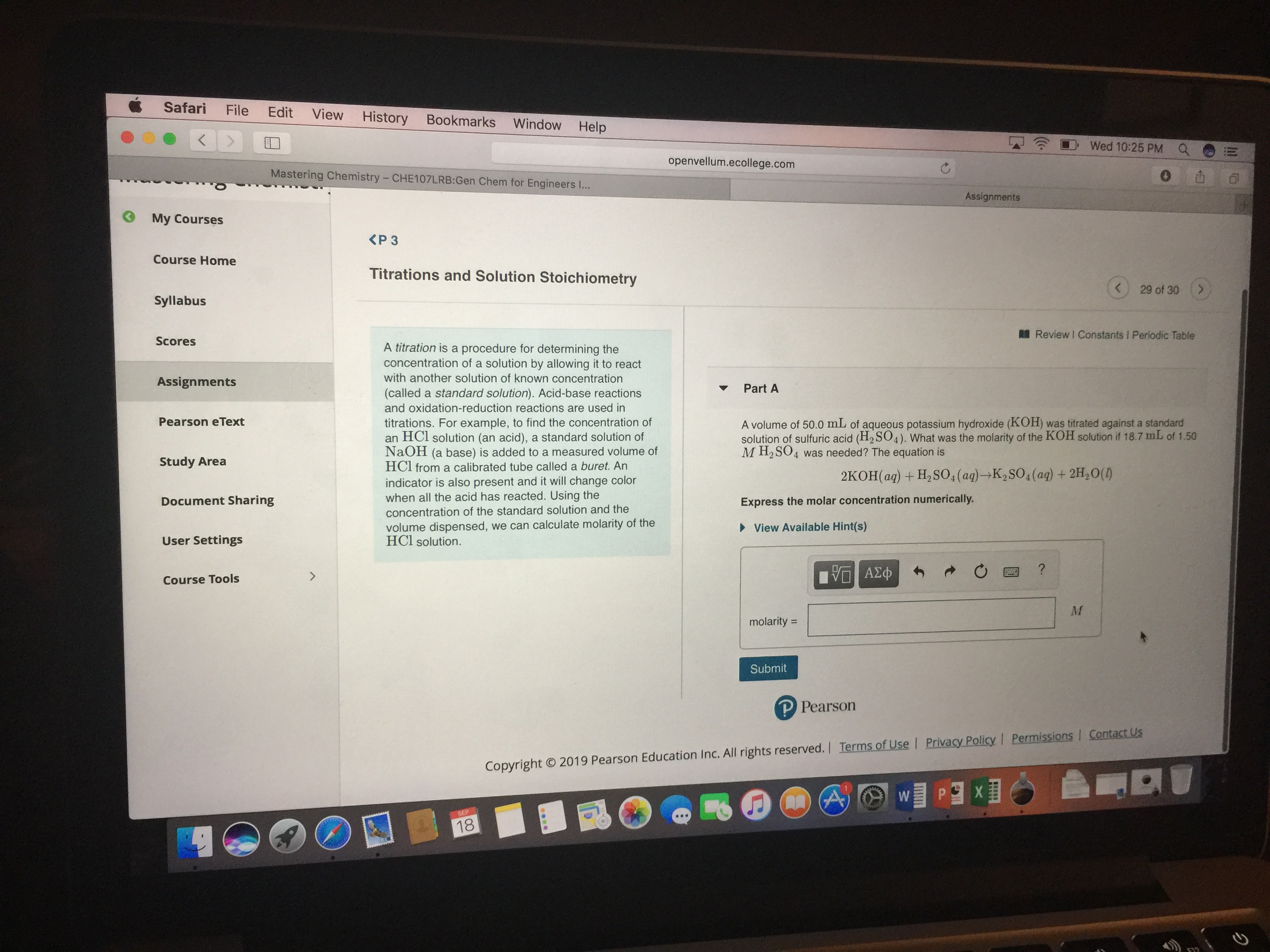# A volume of 50.0 mL of aqueous potassium hydroxide (KOH) was titrated against a standard solution of sulfuric acid (H2SO4). What was the molarity of KOH solution if 18.7 mL of 1.50 M H2SO4 was needed? The equation is: 2KOH(aq) + H2SO4(aq) -> K2SO4(aq) + 2 H2O(l)